Analysis of Variance

The basic idea is to compare alternative estimates of the population variance for the purpose of deciding whether the groups have differnet means. The alternative estimates are predicated on the notion that the mean for certain groups within the population is the same. Under that working supposition there should be no difference in variance estimates constructed from the different sample groups corresponding to the grouping in the population.

One Factor Analysis of Variance

Suppose we have four heads of household, each with a different type of education regarding the effects of alcohol consumption. Their educational program can be regarded as the treatment. For each of them we record their weekly consumption of distilled spirits for each of three weeks. Is their weekly consumption the same? Formally,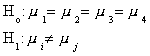We can formulate the problem in one of two alternative ways: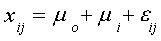i=1,2,3 and j=1,2,3

where i denotes the particular head of household and j denotes the week. In this formulation three heads of household are measured against an arbitrary base person.

An alternative formulation is: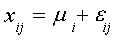i=1,2,3,4 and j=1,2,3

Now each household is measured absolutely.

We have the following data:

 Week Household 1 2 3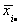x1 13 8 9 10 x2 15 11 13 13 x3 8 12 7 9 x4 11 15 10 12denotes the three week mean for the ith head of household for m=4 households.
The grand mean is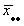= 11.
The sample sizes are n1=n2=n3=n4=3, and n=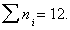.
With respect to the two alternative linear models, the entries in the body of the table are observations on the dependent variable.

If all four households have a common mean, then we could pool the data in the computation of the sample variance as an estimate of the population variance. That is, we could compute the total sum of squares as

SS(TOT)=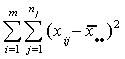(1)

But each household may have a different mean, so using (1) would be in error. Accounting for the differences between households, groups, we would use

SS(E)=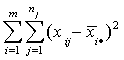(2)

In (2) we compute the sum of squares within a group or treatment, then add them up. This is referred to as the error sum of squares. It is large if there is a lot of variation within the group.

We could also see if there is variation between the groups:
SS(B)=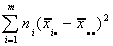This would be large if there is variation between the groups. That is, there are large differences between the group means and the grand mean.

Things to note:

1. Each of the sums of squares is 2 if the variable x is normal with finite mean and variance.

2. Regardless of whether or not the null is true, we know that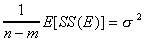, but SS(B) is an unbiased estimator of the population variance only if the group means are equal:

Proof:

E[SS(B)]=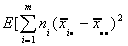=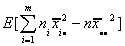Adding and subtracting some terms, we can write this as E[SS(B)]=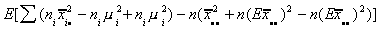=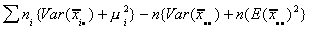=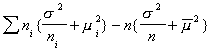=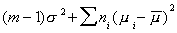This observation suggests the test statistic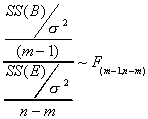m-1 is the number of unique restrictions necessary to impose equality of means across all groups. In the denominator there are n terms in the sum, but we have used the data m times to compute different group means.

For our example:
SS(TOT)=80, SS(E)=50, SS(B)=30

F=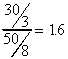Ho is not rejected.

This sort of analysis of variance is often summarized in a table. Almost every computer package has some variant of this general presentation.

 Source Sum of Squares Degrees of Freedom Treatment SS(B) m-1 Error SS(E) n-m Total SS(TOT) n-1

To return to our presentation of the problem as a linear model:

Formulation 1: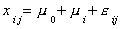With our data and a bit of linear algebra we can write this as: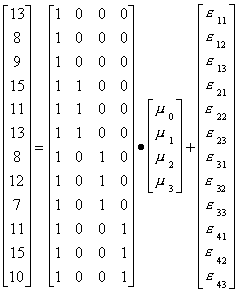Now we can use a regression package to estimate the parameters. The intercept, or mean for household 1 is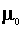. That for household 2 is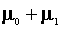. For three it is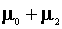and for the last household it is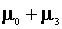. The output for this regression looks like

 Mean of x 11 No. of Obs. 12 R2 0.375 Std. Dev. Of x 2.6968 F(3,8) 1.6 P(F>1.6) 0.26
 Variable Coefficient t-ratio Constant 10.0 6.92 D1 3.0 1.47 D2 -1.0 -.49 D3 2.0 .98

Note:

1. The t-statistics for the coefficients on D1, D2, D3 are not different from zero. That is, none of the group means differ from the mean of the first group.
2. The SS(E)= 50.
3. The F-statistic in the table is 1.6; the same as our previous answer.

Formulation 2: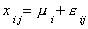With our data and a bit of linear algebra we can write this as: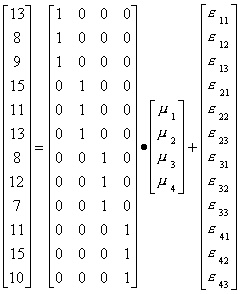And gotten the regression output:

 Variable Coefficient t-ratio D1 10.0 6.928 D2 13.0 9.007 D3 9.0 6.235 D4 12.0 8.314

Note:

1. The top part of the table has not been presented because the information does not change.
2. Now the t-statistics are different from zero. Each of the group means is different from zero, but we don't know if they differ from one another.
3. The F-statistic is again 1.6.

If we fit a linear model with the assumption that the means are equal across groups we get

 Variable Coefficient t-ratio Constant 11.0 14.130

Two Factor Analysis

Suppose that we have four ways of teaching and three different instructors. For the sake of argument, suppose that the standardized test score by class size and teacher is given by:

 Teaching method Instructor Lecture Discussion Computer Video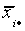1 16 18 21 21 19 2 14 15 18 17 16 3 15 15 18 16 16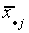15 16 19 18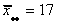Now we believe that the mean test score is affected by instructional method and specific instructor. We could write this as a linear model: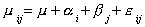where i is the row effect and j is the column effect. Each instructor and method pair is measured relative to the base instructor 1 and lecture method. With this in mind, we have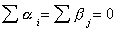, and in the table xij is the mean test score in equal size classes for i=1,2,…,a rows and j=1,2,…,b columns.

The regression results are

 Mean of dependent variable 17 Std. Dev. Of Dependent Var 2.296 R2 0.93 SS(E) 4 F(5,6) 16.2 Pr(F>16.2) 0.002
 Variable Coefficient t-ratio Constant 17.00 29.445 A2 -3.00 -5.196 A3 -3.00 -5.196 B2 1.00 1.500 B3 4.00 6.000 B4 3.00 4.500

where B2: discussion, B3: computer, B4: video and the Aj represent the second and third instructors. The F-statistic indicates that the coefficients are not all zero. In fact, judging from the t-ratios, only the coefficient on B2 is statistically no different from zero.

In general, when there are 'a' rows and 'b' columns, we have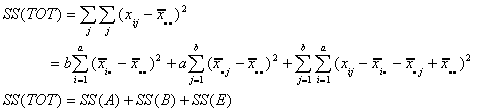So we revise our ANOVA table as

 Source Sum of Squares Deg of Freedom Row Effect SS(A) a-1 Column Effect SS(B) b-1 Error SS(E) (a-1)(b-1) Total SS(TOT) ab-1

To test the row effect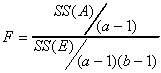To test the column effect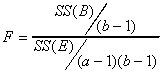For the example

 Source SS df Mean Sq F Row 24 2 12 18 Column 30 3 10 15 Error 4 6 2/3 Total 58 11